## Point Pattern Analysis

Many of the most common kinds of spatial analyses in archaeology involve the assessment of patterning in point-located data where observations represent objects or object attributes linked to XY coordinates in Cartesian space. Data commonly organized in this way include point-plotted artifacts, point-plotted burials, and settlement distributions. With these data, we are often interested in determining the degree to which points (or specific attributes) are clustered, dispersed, or random. Further, we are also often interested in determining how point patterns for different kinds of objects or attributes co-vary or patterns of dispersal and clustering change at different spatial scales.

In this module, I will walk you though a series of useful analyses for conducting basic point-pattern analysis using data from the Paleolithic Liencres site in northern Spain. These data consist of point-plotted artifacts assigned to a number of common artifact categories from a well preserved surface at this open site.

First, let’s read in the point data and the shapefile for the excavation boundary. We can then plot the Excavation boundary with points

``````library(maptools)
library(spatstat)

LiencresEx <- as(LiencresEx, "SpatialPolygons")

Next, we need to convert the point plotted data into a ppp object for the spatstat package that we will be using. This requires us define a boundary (window) for the the points. We’ll use the LiencresEx shapefile as our boundary for now. Following that, we can plot the data.

``````# define the window (class owin) for analyses
W <- as(LiencresEx, "owin")
# Define ppp object based on Liencres point locations
Liencres.pp <- ppp(x = Liencres\$E, y = Liencres\$N, window = W, marks = Liencres\$Name)
# now let's plot it
plot(Liencres.pp, cex = 0.5)``````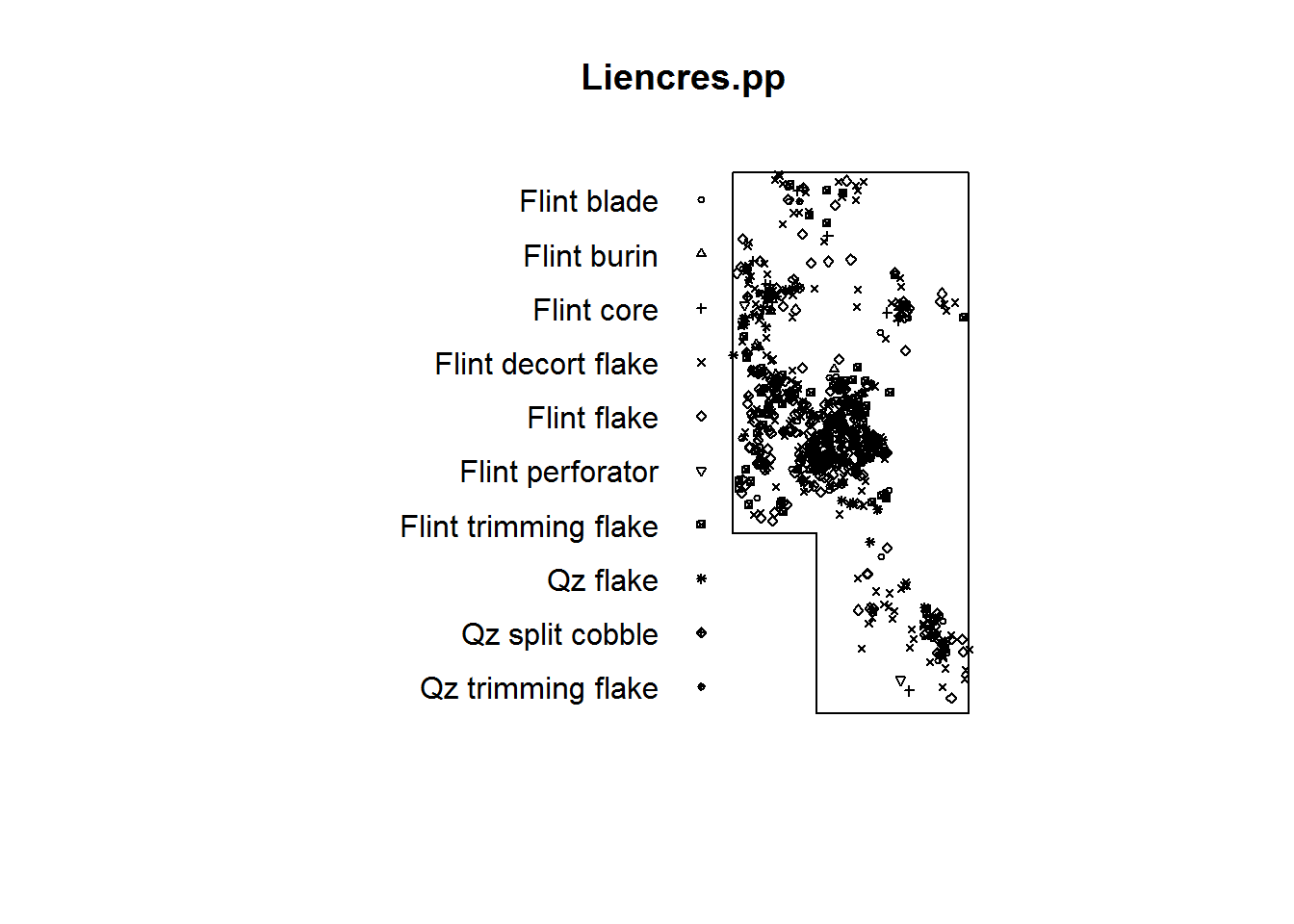Okay, now let’s calculate the Ripley’s K function for all points at this site and plot the results. Ripley’s K is a method of determining whether a point pattern deviates from complete spatial randomness (CSR) at a given distance. We’ll use the Kest function from the spatstat package. In this example, we’re using the “Ripley” correction factor that helps us deal with edge effects. See help(Kest) for more info.

``````# calculate and plot Ripley's K function using the 'Ripley' correction
kf <- Kest(Liencres.pp, correction = "Ripley")
plot(kf)``````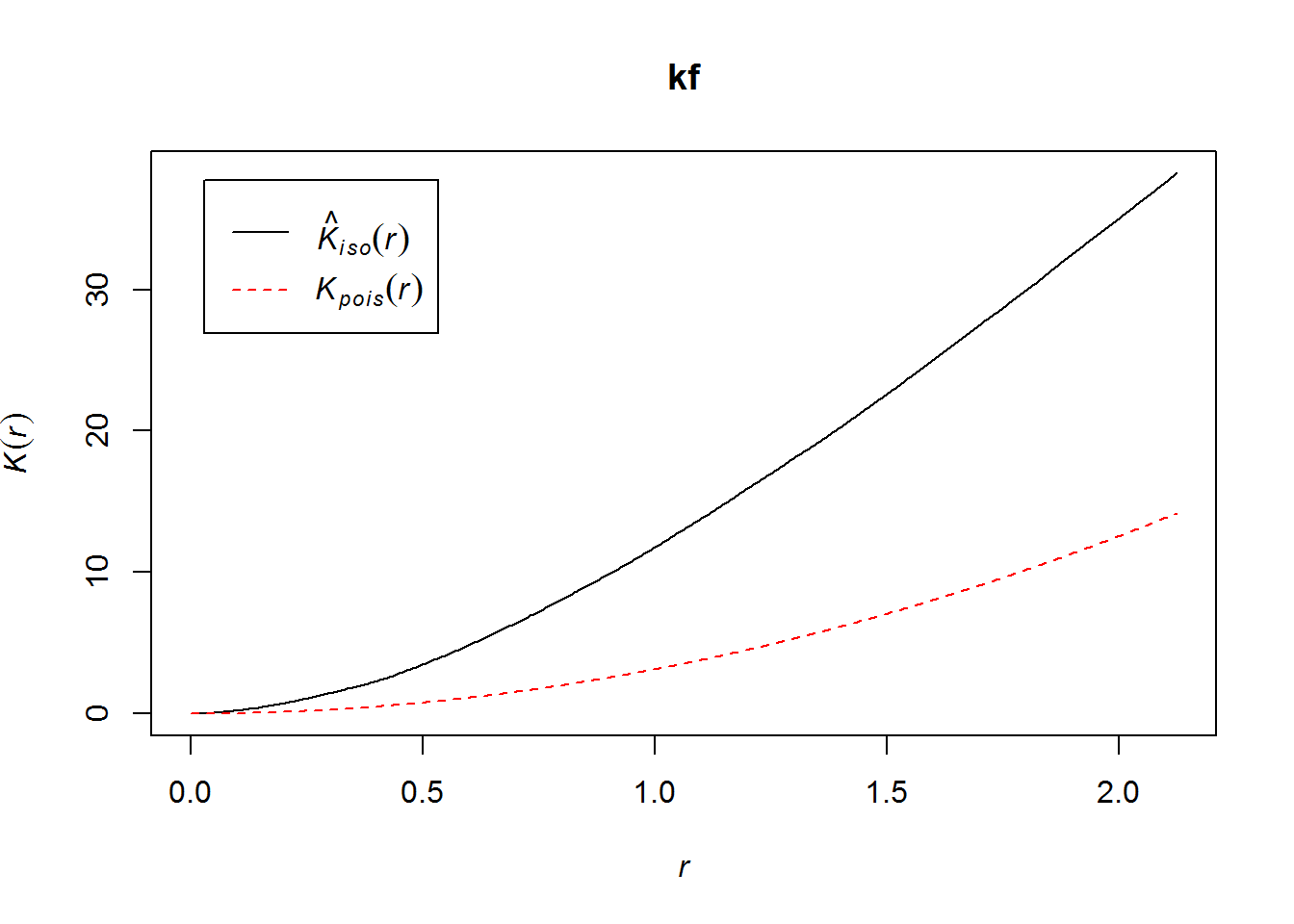The plot shows that at all tested distances the actual observed value of K-hat is greater than the theoretically derived expected value indicating clustering.

In many cases, it is useful to simulate the potential effects of sampling by creating what is called an “envelope” from the actual data. Specifically, this procedure involves simulating some number of datasets by permuting the locations of input data (99 times by default) and then obtaining the range of K-hat values across a range of distances for each permuted dataset. We can then plot the K estimates at each distance for the original data along with the “envelope” showing the range for the simulated datasets. When the sample size is high, the envelope will tend to be small.

``````# calculate envelope around K-hat estimates. The arguement verbose=F just
# keeps the output from showing progress as the command runs.
kf.env <- envelope(Liencres.pp, Kest, correction = "Ripley", verbose = F)
plot(kf.env)``````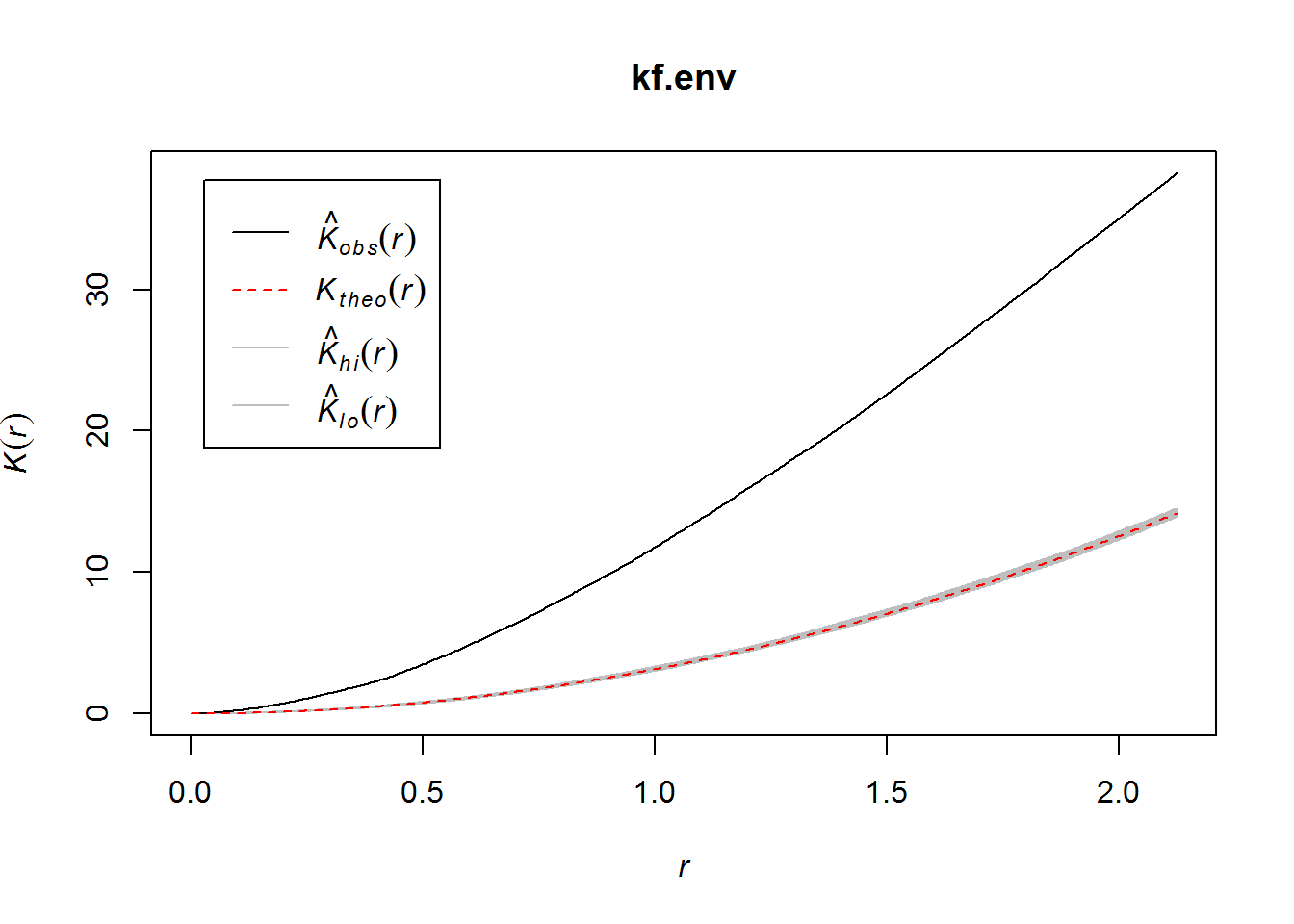Once again, this plot shows that K-hat is considerably higher than the envelope of simulated K values across all but the shortest distances suggesting significant clustering and deviations from CSR.

In many cases, it is preferable to use a modified version of Ripley’s K known as the L function. L is simply the square root of K at a given distance divided by pi. This essentially stabilizes K by the variance, often producing more interpretable results. It is possible to calculate L just like K using the envelope method described above.

``````# calculate envelope around L-hat estimates.
lf.env <- envelope(Liencres.pp, Lest, correction = "Ripley", verbose = F)
plot(lf.env)``````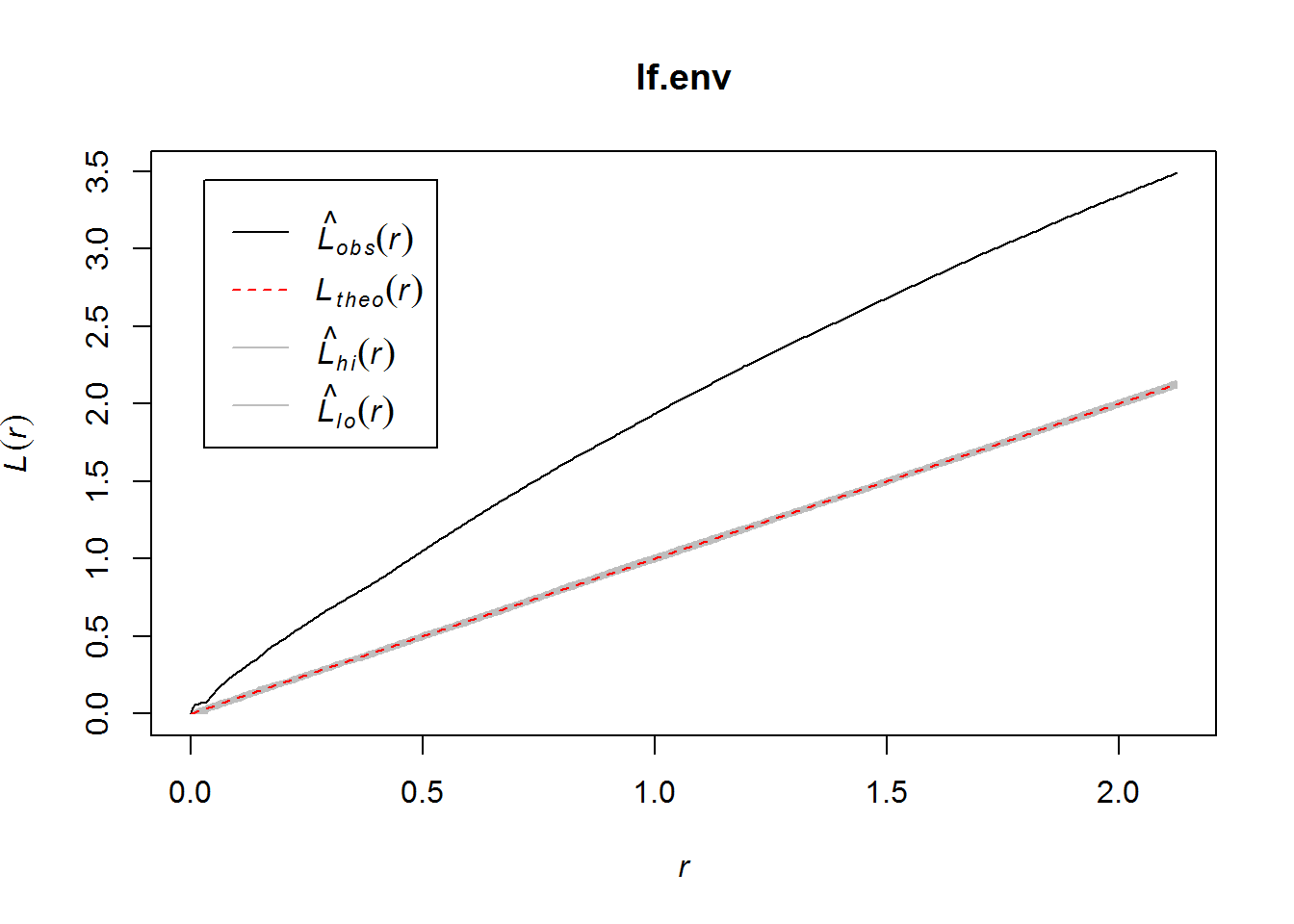The values of L-hat once again demonstrate considerable clustering at all distances.

It is often further helpful to recenter values of L-hat in these plots by subtracting r at each distance so that L-hat is always defined as 0 at all distances and deviations of L-hat from L are shown in relation to this horizontal line. In the plots below, values above the line indicate clustering and values below the line indicate dispersion.

Note that some packages calculate this recentered version of L as r-L rather than L-r as we’re doing here. For example the PASSaGE 2.0 software that Stojanowski used in your reading for this week calculates r-L so that deviations above line line indicate dispersion and deviations below the line indicate clustering. There seem to be preferences for one or the other equation in different fields but the decision is really arbitrary. Make sure you always check documentation carefully to know which equation your software package uses.

``````# plot recentered L results
plot(lf.env, . - r ~ r)``````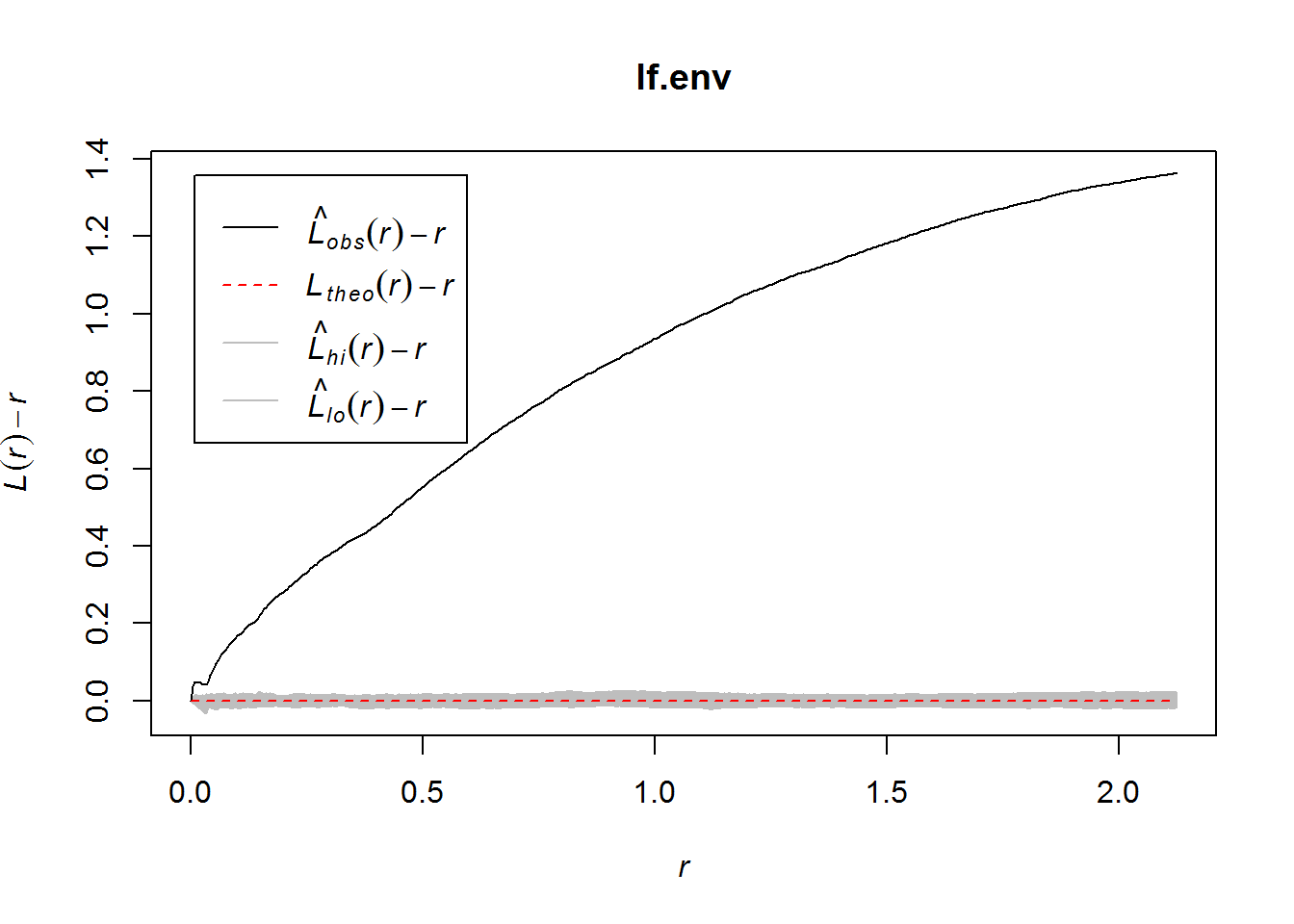Importantly, we can also calculate K and L functions for individual artifact classes using the “marks” argument we defined above.

``````# calculate L-hat for the Flint core mark only
lf.mark.env <- envelope(Liencres.pp[Liencres.pp\$marks == "Flint core"], Lest,
correction = "Ripley", verbose = F)
plot(lf.mark.env, . - r ~ r)``````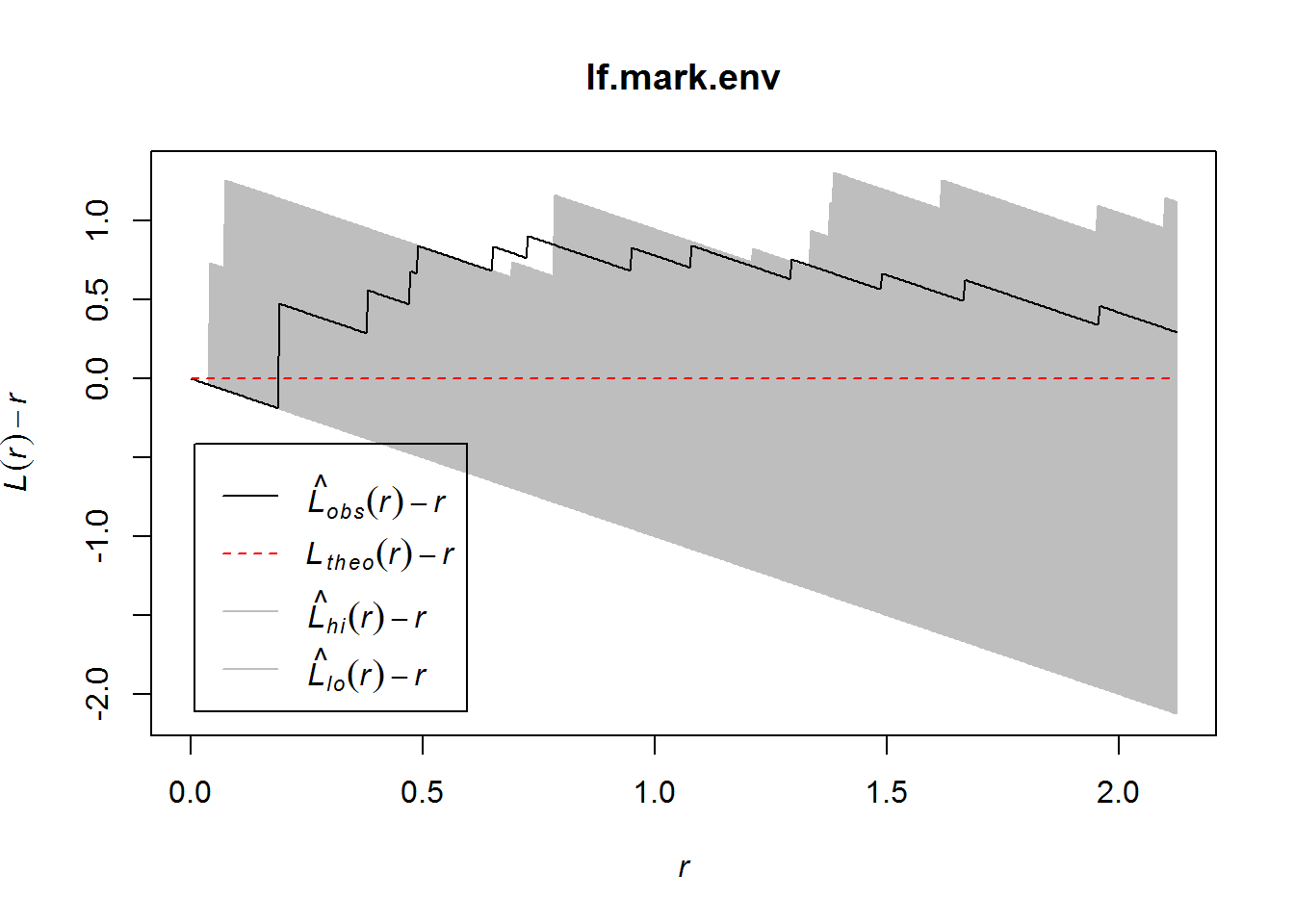As this plot shows, the value of L-hat for Flint cores is within the envelope of simulated values of L for most distances suggesting that this particular artifact category is not considerably more clustered than we might expect by chance given the total number of cores present.

It is also possible to compare two “mark” categories using either the K or L functions using the Kcross or Lcross command respectively. These commands both take two mark names as arguments (denoted as i and j) and determine the degree to which mark j is clustered or dispersed from mark i. The argument i always indicates the type of point from which distances are measured and j indicates the type to which distances are measured. Let’s try an example again using the envelope function.

``````# Calculate L-hat between Qz flakes and Qz split cobbles
lf.cross <- envelope(Liencres.pp, Lcross, i = "Qz flake", j = "Qz split cobble",
verbose = F)
plot(lf.cross, . - r ~ r)``````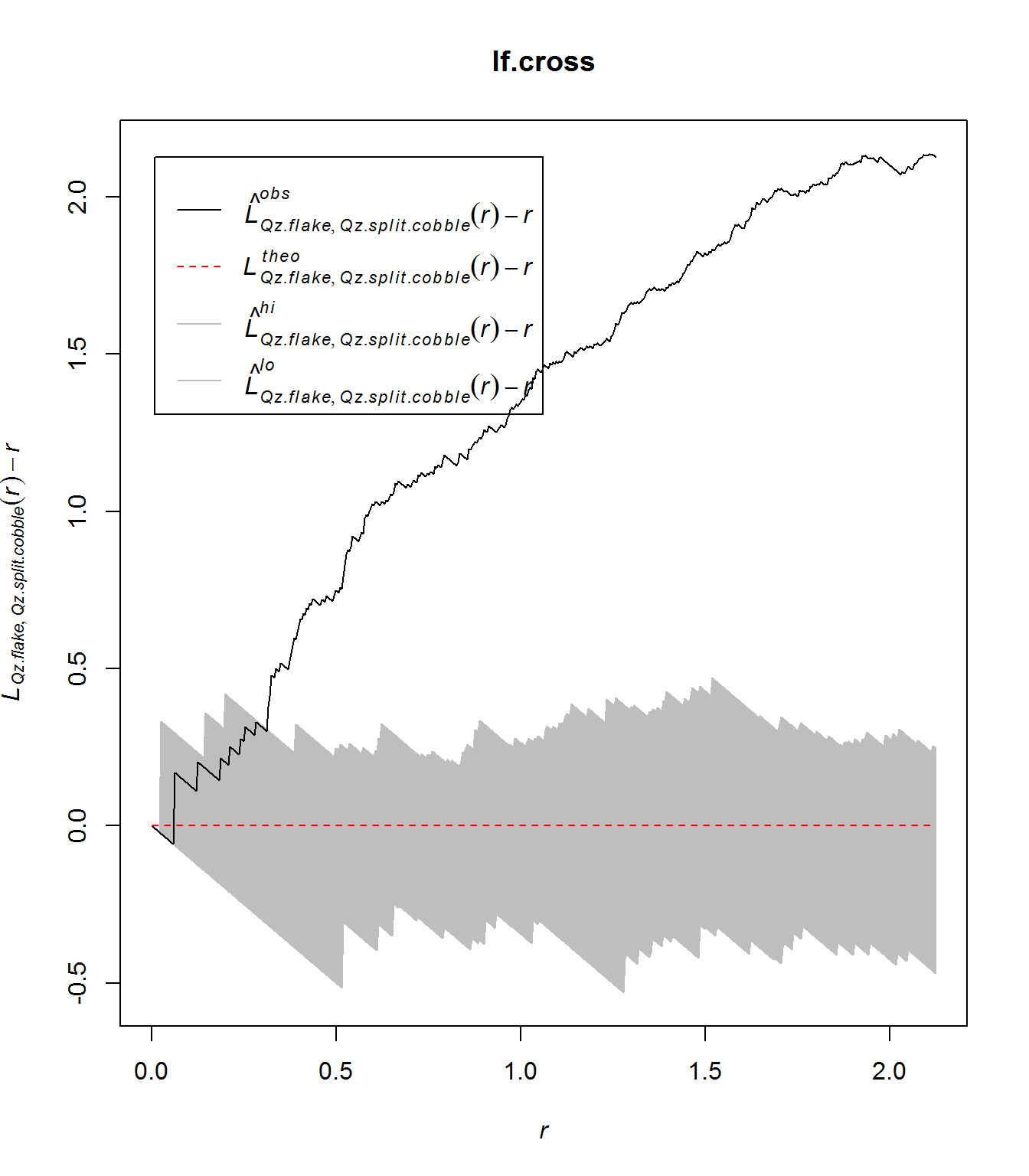In this example, Qz split cobbles are non-randomly clustered in relation to Qz flakes, but only when distance beyond about 0.3 meters are considered.

We can also calculate Kdot and Ldot which calcuate the relationship between mark i and all other types in a dataset. Here is a quick example.

``````# Calculate L-hat between Flint decortication flakes and all other artifact
# categories
lf.dot <- envelope(Liencres.pp, Ldot, i = "Flint decort flake", verbose = F)
plot(lf.dot, . - r ~ r)``````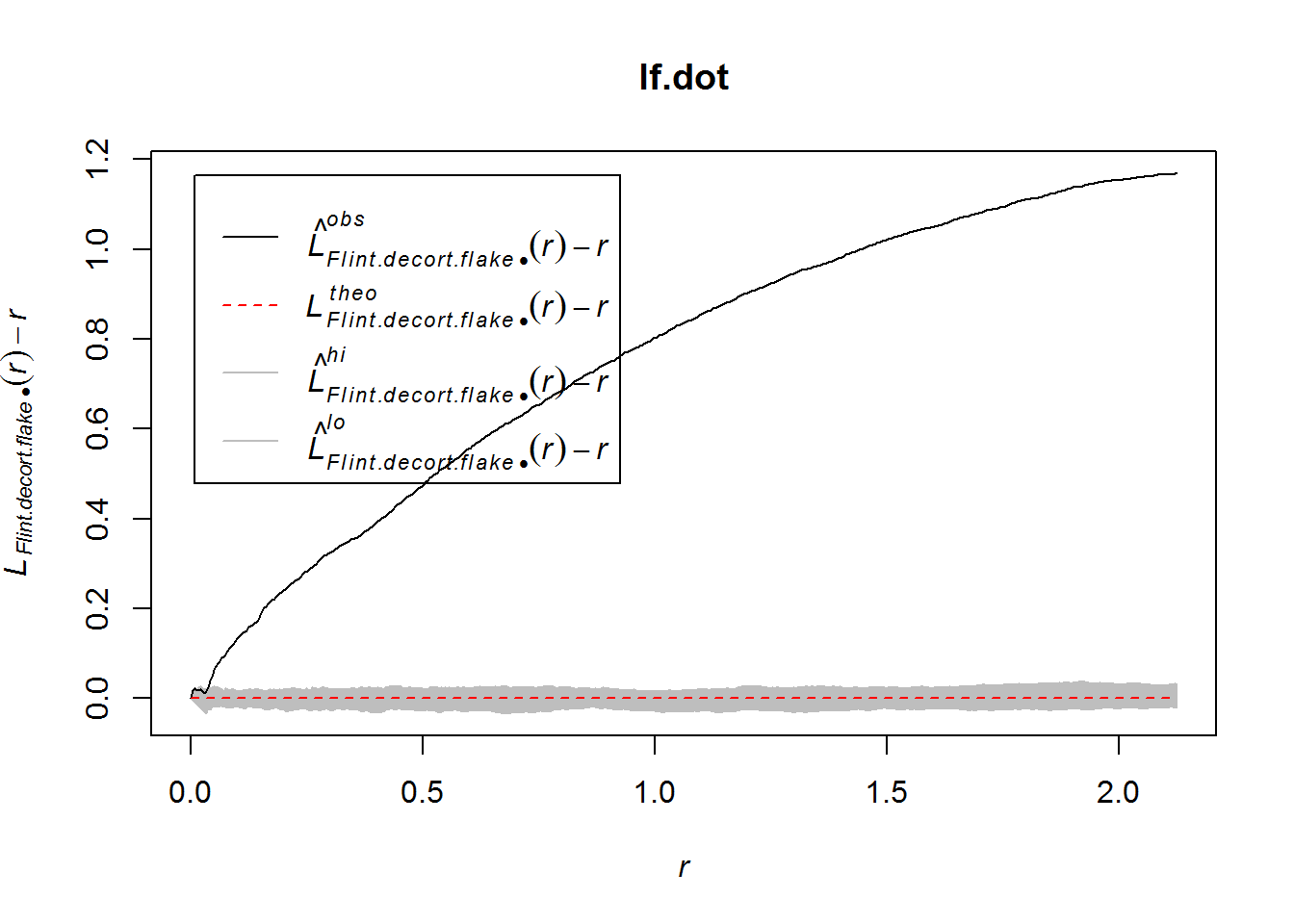Not surprisingly, flint decortication flakes are significantly clustered at all consdered distances.

## Dealing with inhomogeneous data

So far, all of the examples we have shown have assumed an homogeneous Poisson point process, meaning that we are assuming that the locations of points across the site are independent (a Poisson process) and that they DO NOT differ in intensity from place to place (homogeneous). An inhomogeneous Poisson point process refers to a distribution generated by process where the locations of points across the site are independent but they DO differ in intensity. How can we tell if our point distribution is inhomogeneous? In many cases we can simply create a density map of all points and assess the degree to which there are concentrated areas of high density. Let’s do that for the Liencres data. The value for sigma below defines the interpolation scale for the density plot. I’ve provided three values to give you an idea of how this can impact your assessments of density.

``````par(mfrow = c(1, 3))  # set up to plot 3 plots on one row
# plot density at various levels of sigma
plot(density(Liencres.pp, sigma = 0.5), main = "Sigma 0.5")
plot(density(Liencres.pp, sigma = 0.75), main = "Sigma 0.75")
plot(density(Liencres.pp, sigma = 1), main = "Sigma 1.0")``````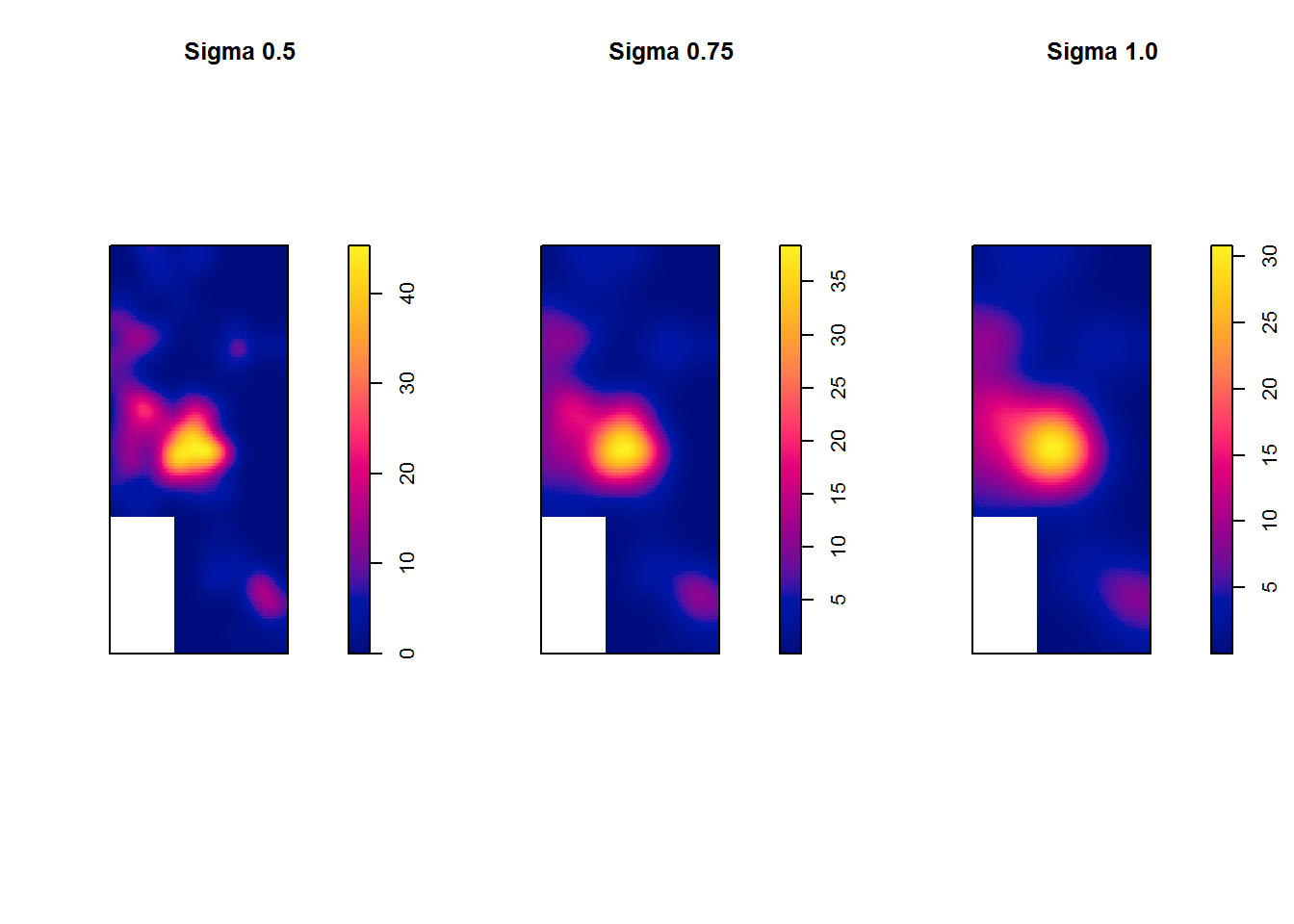The data in the Liencres example are pretty clearly inhomogeneous, but it isn’t always so easy to tell. Another method sometimes used assess the homogeneity of Poisson point processes is known as a quadrat test. Essentially, a grid is placed across the point patterns and a chi-square test is used to assess the degree to which the grid counts differ from expected. We determine the size of the grid squares by specifying the number of cells in both the x and y dimensions. This decision should be informed by the scale at which we wish to assess homogeneity.

``````# create a quardat test statistic by simulating the p-value using Monte
# Carlo methods to help deal with cells with low counts. In this example,
# nx=4,ny=10 means that we will create a grid 4 squares wide and 10 high (2
# meter resolution in this case)
set.seed(324324)  # set random seed for repeatability
(M <- quadrat.test(Liencres.pp, nx = 4, ny = 10, method = "MonteCarlo"))``````
``````##
##  Conditional Monte Carlo test of CSR using quadrat counts
##  Pearson X2 statistic
##
## data:  Liencres.pp
## X2 = 1370.8, p-value = 0.001
## alternative hypothesis: two.sided
##
## Quadrats: 37 tiles (irregular windows)``````

In this example we get a statistically significant result suggest that the observed quadrats differ from the expected under a homogeneous Poisson point process model. It is sometimes useful to display the quadrat counts directly. In the code below I plot observed and expected quadrat counts on a density map of the Liencres data. In this plot for each grid square the observed count is shown in the top left, the expected is shown in the top right, and the Pearson residual is shown at the bottom. Residuals are calculated as (observed-expected)/sqrt(expected) such that the residuals have a mean of 0 and variance of 1 meaning that deviations far beyond -1 to 1 in either direction are important where as variables within the range of -1 to 1 are expected.

``````# plot density map and display grid info
plot(density(Liencres.pp), main = "Grid Counts")
plot(M, cex = 0.5, col = "white", add = T)``````